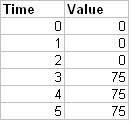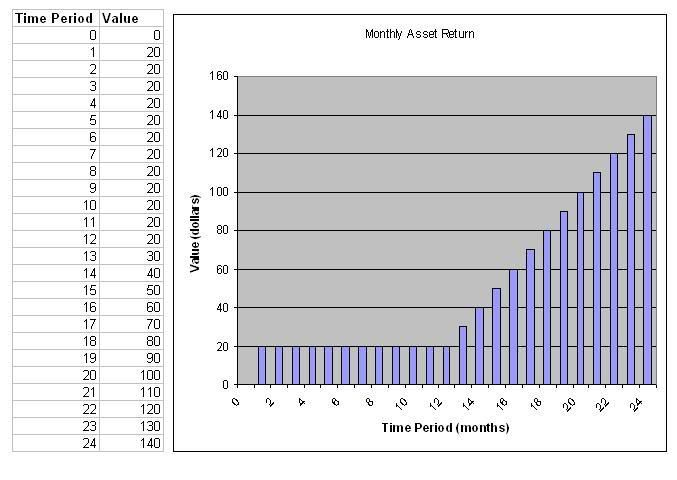Example Time Shift

# Example

Example 1. Suppose an asset will provide nothing until the 3rd month, when it provides 75 dollars a month until month 5. The monthly interest rate is 9%.1. Use time shifting to find the present value of this asset.

The present worth of this asset is it's value in time period zero. To find this, we need to take the value at time periods 3 through 5 and use time shifting to move it to time period zero. Note that the 3 in the factor notation represents how many values are being shifted. The 2 in the exponent of the time shifting equation represents the number of time periods being shifted.

Present Worth=75(P|A,i=.09,N=3)/(1+.09)2

Example 2. Suppose an asset provides \$20 per month after the first month. This continues until month 13, when the asset begins to increase by \$10 a month until month 24. The monthly interest rate is 6%.1. Using factor notation, how would you represent the present value of these benefits after 24 months?

It is important to note here that this is a decomposition problem of a constant series combined with a linear gradient series. We need to shift the linear gradient series back to time period zero to get the present value of both series. The periods zero and one in a linear gradient series must have a value of zero, so we know that the series begins in time period 11. We shift the linear gradient series back 11 time periods to time period zero to get the present value. We also need to add the present value of the constant series.

P=20(P|A, i=.06, 24) + 10(P|G, i=.06, 13)(1+.06)-11

2. Using factor notation, how would you represent the present value of the benefits between months 5 and 12?

Here we pick up on a constant series from month 5 to month 12. The first time period in a constant series must have a value of zero, so we know that the series begins in time period 4. We must shift this series ahead 4 time periods from time period zero to time period 4 to get the present value of the benefits between months 5 and 12.

P=20(P|A, i=.06, 8)(1+.06)-4

page revision: 3, last edited: 27 Jun 2016 17:06